Latest Banking jobs   »   NIACL AO 2021 Result Out, Mains Exam Date For 300 AO Recruitment   »   Quantitative Aptitude Quiz For IBPS Clerk/NIACL...

# Quantitative Aptitude Quiz For IBPS Clerk/NIACL AO Prelims 2021- 13th September

Directions (1-5): In each of these questions, two equations (I) and (II) are given. You have to solve both the equations and give answer
(a) if x>y
(b) if x≥y
(c) if x<y
(d) if x ≤y
(e) if x = y or no relation can be established between x and y.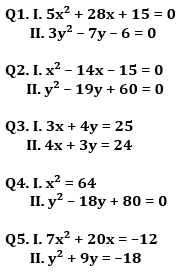Directions (6-10): In each of these questions, two equations (i) and (ii) are given. You have to solve both the equations and give answer
(a) if x>y
(b) if x≥y
(c) if x<y
(d) if x ≤y
(e) if x = y or no relation can be established between x and y.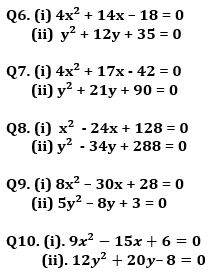Directions (11-15): In the following questions, two equations (I) and (II) are given. You have to solve both the equations and mark the appropriate answer.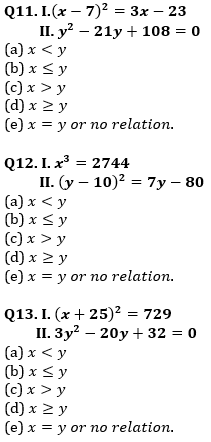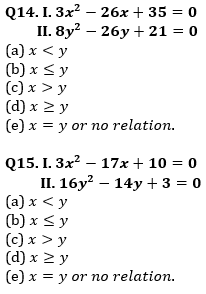Solutions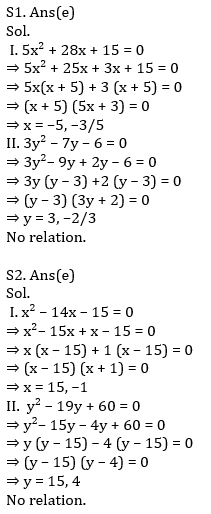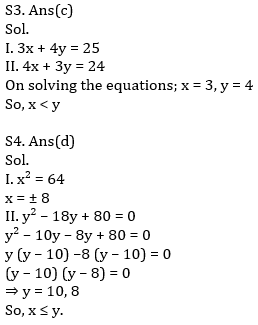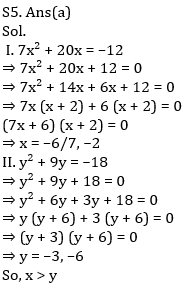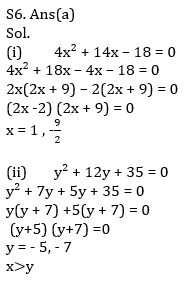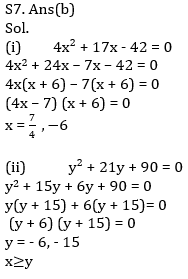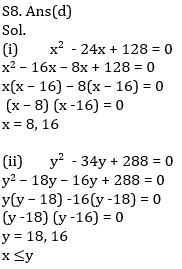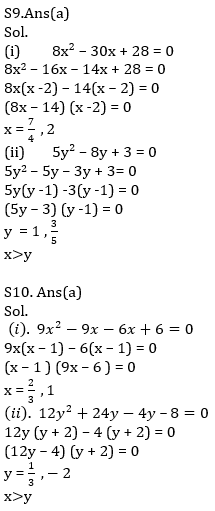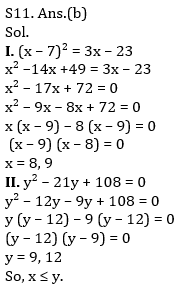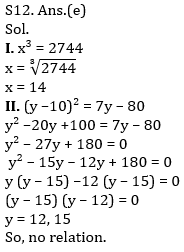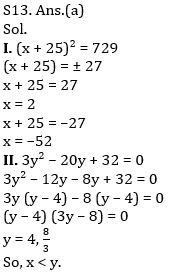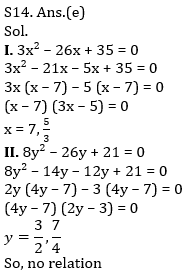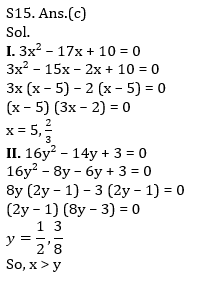Click Here to Register for Bank Exams 2021 Preparation Material#### Congratulations!Download Hindu Review of October 2021: Free PDF